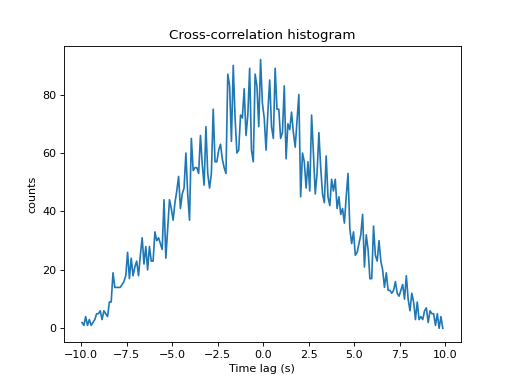# viziphant.spike_train_correlation.plot_cross_correlation_histogram¶

viziphant.spike_train_correlation.plot_cross_correlation_histogram(cch, axes=None, units=None, maxlag=None, legend=None, title='Cross-correlation histogram')[source]

Plot a cross-correlation histogram returned by `elephant.spike_train_correlation.cross_correlation_histogram()`, rescaled to seconds.

Parameters
cchneo.AnalogSignal or list of neo.AnalogSignal

Cross-correlation histogram or a list of such.

axesmatplotlib.axes.Axes or None, optional

Matplotlib axes handle. If set to None, new axes are created and returned. Default: None

unitspq.Quantity or str or None, optional

Desired time axis units. If None, `cch.sampling_period` units are used. Default: None

maxlagpq.Quantity or None, optional

Left and right borders of the plot. Default: None

legendstr or list of str or None, optional

The axes legend labels. Default: None

titlestr, optional

The axes title. Default: ‘Cross-correlation histogram’

Returns
figmatplotlib.figure.Figure
axmatplotlib.axes.Axes

Examples

```import quantities as pq
import matplotlib.pyplot as plt
from elephant.spike_train_generation import homogeneous_poisson_process
from elephant.conversion import BinnedSpikeTrain
from elephant.spike_train_correlation import              cross_correlation_histogram
from viziphant.spike_train_correlation import              plot_cross_correlation_histogram

spiketrain1 = homogeneous_poisson_process(rate=10*pq.Hz,
t_stop=10*pq.s)
spiketrain2 = homogeneous_poisson_process(rate=10*pq.Hz,
t_stop=10*pq.s)
binned_spiketrain1 = BinnedSpikeTrain(spiketrain1, bin_size=100*pq.ms)
binned_spiketrain2 = BinnedSpikeTrain(spiketrain2, bin_size=100*pq.ms)
cch, lags = cross_correlation_histogram(binned_spiketrain1,
binned_spiketrain2)

plot_cross_correlation_histogram(cch)
plt.show()
```